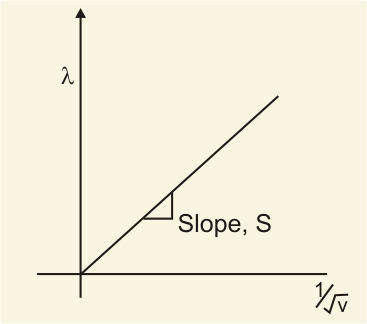# Plot a graph showing variation of de-Broglie wavelength  associated with a charged particle mass , versus ,Where  is the potential difference through which the particle is accelerated. How does this graph give us the information regarding the magnitude of the charge of the particle

Let us consider a particle of charge  and mass

To gain speed  and kinetic energy  Let it be accelerated by a potential difference

Thus,

Now, on Rearranging the expression, we have

The de-Broglie wavelength equation :-

On substituting the value of  we have

Now, we know the equation of straight line.-

Where  is the shape of the line.

Hence, on plotting the graph of  vs .

We will have a straight line with a slope.

On rearranging, we have

The graph of  vs . Can be drawn as :Hence, we can determine  from the graph, and this graph gives us the information regarding the magnitude of the charge of the particle.

## Related Chapters

### Preparation Products

##### Knockout KCET 2021

An exhaustive E-learning program for the complete preparation of KCET exam..

₹ 4999/- ₹ 2999/-
##### Knockout KCET JEE Main 2021

It is an exhaustive preparation module made exclusively for cracking JEE & KCET.

₹ 27999/- ₹ 16999/-
##### Knockout NEET Sept 2020

An exhaustive E-learning program for the complete preparation of NEET..

₹ 15999/- ₹ 6999/-
##### Rank Booster NEET 2020

This course will help student to be better prepared and study in the right direction for NEET..

₹ 9999/- ₹ 4999/-